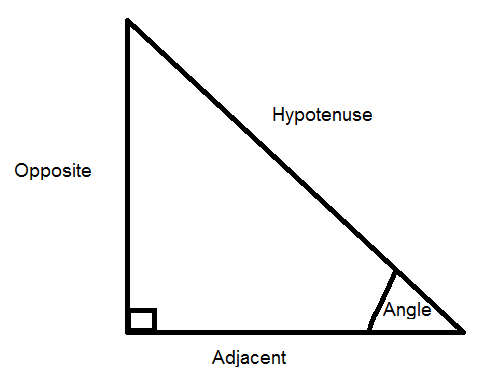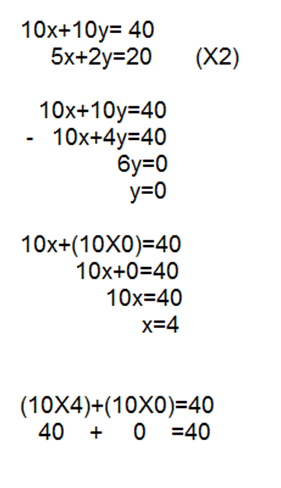List of public pages created with Protopage

# Home

## Plain sticky notes

### Intro

This is my page where I will be writing stuff about GCSE maths exams, Unit A,B and C Hope it helps :)

# Unit A

## Plain sticky notes

### Info

This exam is a calculator exam and will contain things like Trigonometry, Pythagorus' Theoram,Loci, Algebra and Histograms.

### Loci

Loci is basically a line that shows all the points that fit in with a given rule. Here are 2 important rules: 1.The locus of a point which is a fixed distance from a point is simply a circle around it 2.The locus of a straight line is basically a rectangle around it but instead of the 2 short edges you have 2 curved ones to make it look like an oval

### Trigonometry

Here is where the SohCahToa song comes in handy above. In this you have a RIGHT ANGLED triangle and you have to work out 1 of the lengths if you are given another 1 and an angle WITHOUT measuring it! Firstly you need to label the 3 sides, the opposite (O), the adjacent (A) and the hypotenuse (H). The H is always opposite the right angle, and the other 2 sides depend on where the angle is. The opposite is always opposite the angle so the adjacent is the last one left. Then you need to learn the fomula triangles that spell SohCahToa and MEMORISE them then it is easy.

## Rich sticky notes

### Trigonometry Example# Unit B

## Plain sticky notes

### Info

This is a non calculator exam and it will contain things like Surds, Vectors, Graphs, Simultaneous Equations and Circle Theorams.

### Simultaneous Equations

Rules: 1. Make sure the number before either the X or Y is the same if not then make them the same by multiplying to get one of them the same ( negatives don't matter at this stage) 2. If the signs are the same before the one you are using (either X or Y) then you subtract the 2 equations and if they are opposite then add them 3. Rearrange to find the value of either X or Y (e.g. 10x= 20 so X=2) 4. Substitute in the value of X or Y to find the second value, you will end up with X= something and Y=something. 5. The to check it put the values back into the equation and check you get the same answer.

## Rich sticky notes

### Simultaneous Equations Example# Unit C

## Plain sticky notes

### Info

This exam is a calucator exam and contains part of Unit A, Unit B and extra. Examples of things in this is Algebra, Equations, Probability, Triangles and Graphs.